# NYS COMMON CORE MATHEMATICS CURRICULUM A Story of

• Slides: 65
Download presentationNYS COMMON CORE MATHEMATICS CURRICULUM A Story of Ratios Grade 8 – Module 2 © 2012 Common Core, Inc. All rights reserved. commoncore. orgNYS COMMON CORE MATHEMATICS CURRICULUM A Story of Ratios Session Objectives • Examine the development of mathematical understanding across the module using a focus on concept development within the lessons. • Identify the big idea within each topic in order to support instructional choices that achieve the lesson objectives while maintaining rigor within the curriculum. © 2012 Common Core, Inc. All rights reserved. commoncore. orgNYS COMMON CORE MATHEMATICS CURRICULUM Agenda Introduction to the Module Concept Development Module Review © 2012 Common Core, Inc. All rights reserved. commoncore. org A Story of Ratios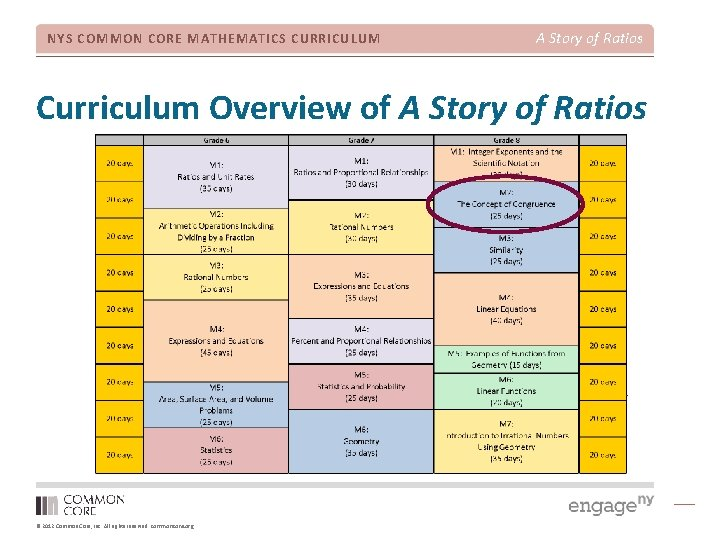NYS COMMON CORE MATHEMATICS CURRICULUM A Story of Ratios Curriculum Overview of A Story of Ratios © 2012 Common Core, Inc. All rights reserved. commoncore. org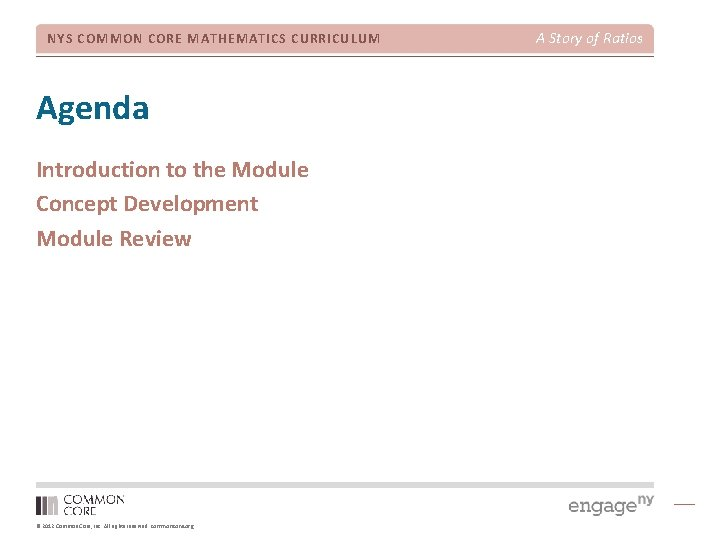NYS COMMON CORE MATHEMATICS CURRICULUM Agenda Introduction to the Module Concept Development Module Review © 2012 Common Core, Inc. All rights reserved. commoncore. org A Story of Ratios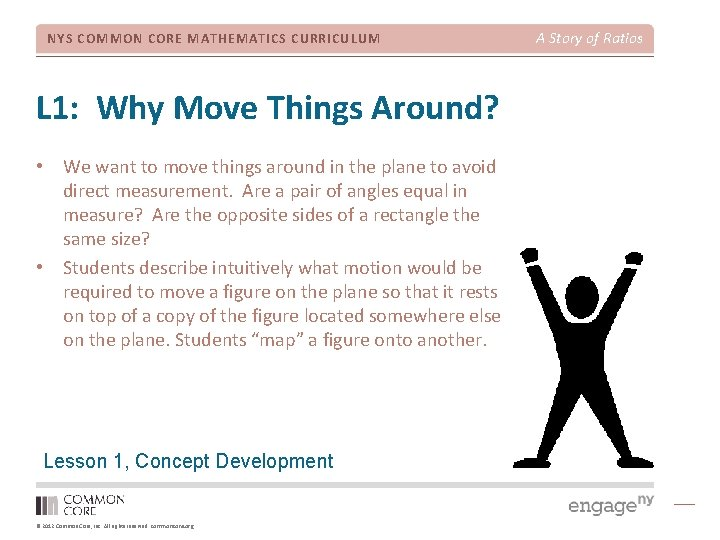NYS COMMON CORE MATHEMATICS CURRICULUM L 1: Why Move Things Around? • We want to move things around in the plane to avoid direct measurement. Are a pair of angles equal in measure? Are the opposite sides of a rectangle the same size? • Students describe intuitively what motion would be required to move a figure on the plane so that it rests on top of a copy of the figure located somewhere else on the plane. Students “map” a figure onto another. Lesson 1, Concept Development © 2012 Common Core, Inc. All rights reserved. commoncore. org A Story of RatiosNYS COMMON CORE MATHEMATICS CURRICULUM A Story of Ratios Exploratory Challenge 1 Slide the original figure to the image (1) until they coincide. Slide the original figure to (2), then flip it so they coincide. Slide the original figure to (3), then turn it until they coincide. © 2012 Common Core, Inc. All rights reserved. commoncore. org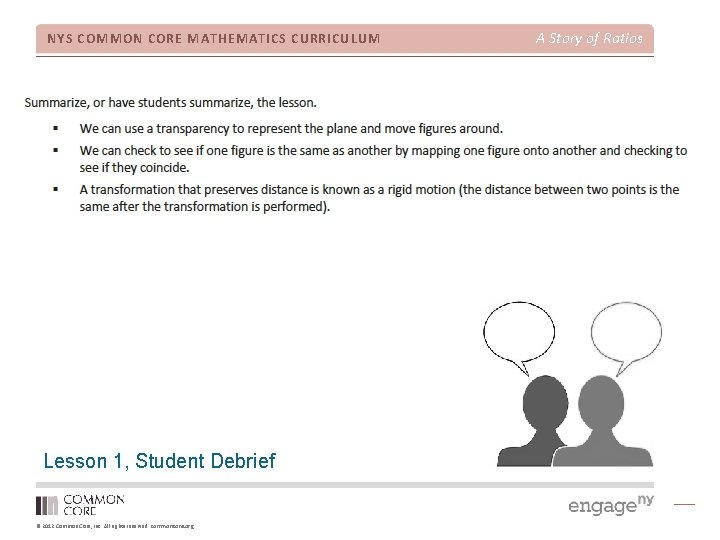NYS COMMON CORE MATHEMATICS CURRICULUM Lesson 1, Student Debrief © 2012 Common Core, Inc. All rights reserved. commoncore. org A Story of Ratios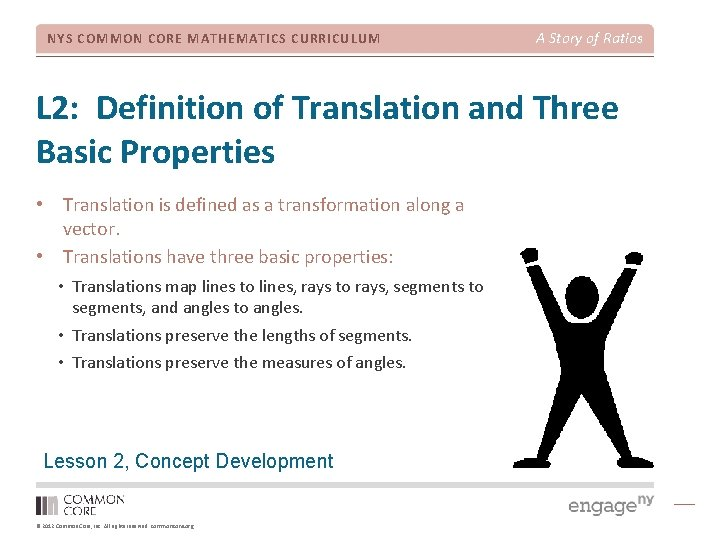NYS COMMON CORE MATHEMATICS CURRICULUM A Story of Ratios L 2: Definition of Translation and Three Basic Properties • Translation is defined as a transformation along a vector. • Translations have three basic properties: • Translations map lines to lines, rays to rays, segments to segments, and angles to angles. • Translations preserve the lengths of segments. • Translations preserve the measures of angles. Lesson 2, Concept Development © 2012 Common Core, Inc. All rights reserved. commoncore. org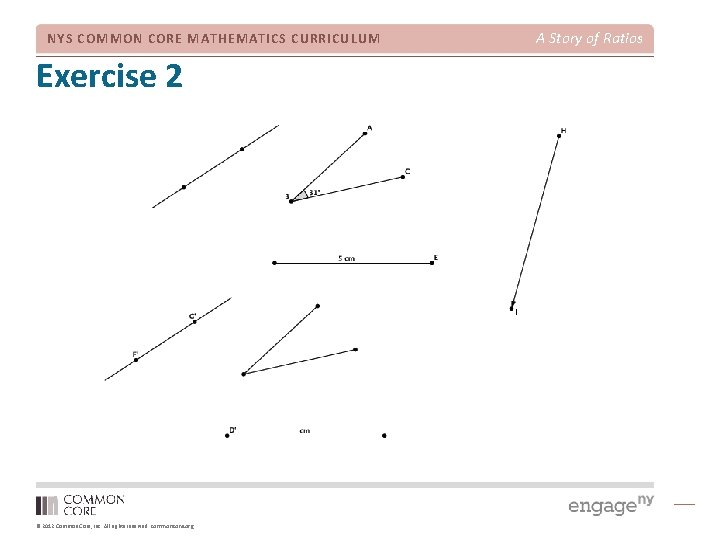NYS COMMON CORE MATHEMATICS CURRICULUM Exercise 2 © 2012 Common Core, Inc. All rights reserved. commoncore. org A Story of Ratios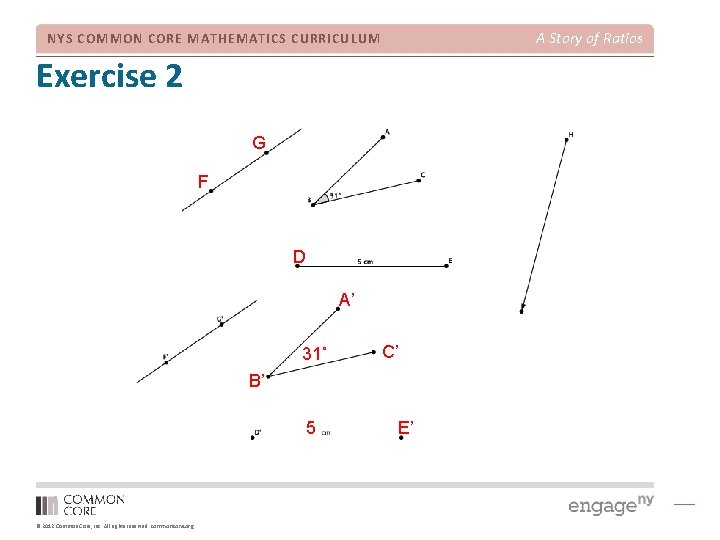A Story of Ratios NYS COMMON CORE MATHEMATICS CURRICULUM Exercise 2 G F D A’ 31˚ C’ B’ 5 © 2012 Common Core, Inc. All rights reserved. commoncore. org E’NYS COMMON CORE MATHEMATICS CURRICULUM Lesson 2, Student Debrief © 2012 Common Core, Inc. All rights reserved. commoncore. org A Story of Ratios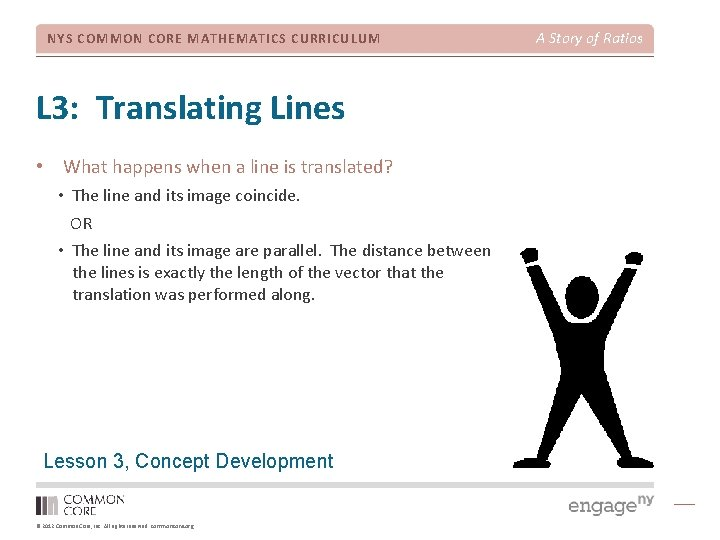NYS COMMON CORE MATHEMATICS CURRICULUM L 3: Translating Lines • What happens when a line is translated? • The line and its image coincide. OR • The line and its image are parallel. The distance between the lines is exactly the length of the vector that the translation was performed along. Lesson 3, Concept Development © 2012 Common Core, Inc. All rights reserved. commoncore. org A Story of RatiosNYS COMMON CORE MATHEMATICS CURRICULUM Exercises 1 -4 © 2012 Common Core, Inc. All rights reserved. commoncore. org A Story of RatiosNYS COMMON CORE MATHEMATICS CURRICULUM Exercises 1 -4 © 2012 Common Core, Inc. All rights reserved. commoncore. org A Story of RatiosNYS COMMON CORE MATHEMATICS CURRICULUM Exercises 1 -4 © 2012 Common Core, Inc. All rights reserved. commoncore. org A Story of Ratios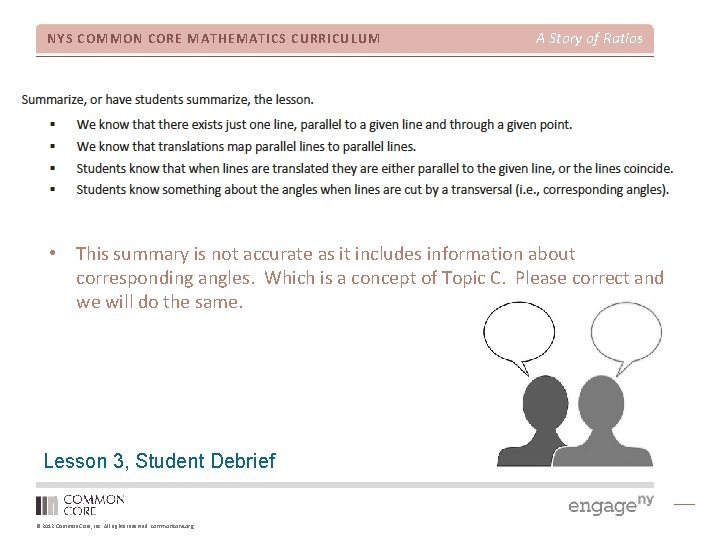NYS COMMON CORE MATHEMATICS CURRICULUM A Story of Ratios • This summary is not accurate as it includes information about corresponding angles. Which is a concept of Topic C. Please correct and we will do the same. Lesson 3, Student Debrief © 2012 Common Core, Inc. All rights reserved. commoncore. org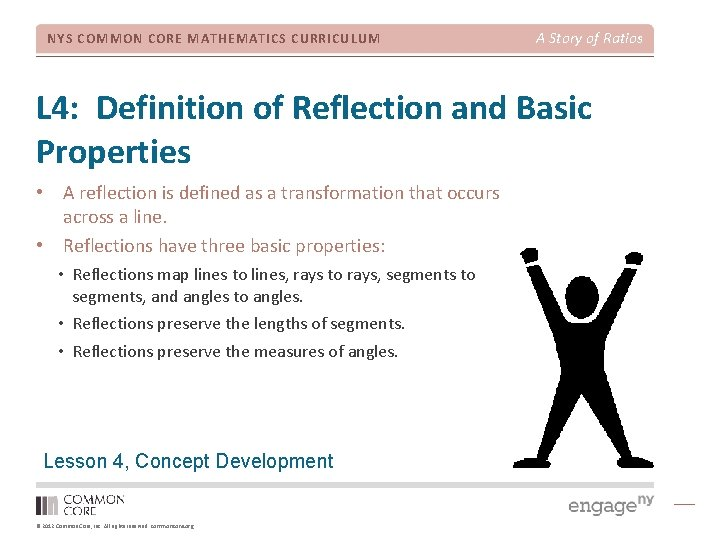NYS COMMON CORE MATHEMATICS CURRICULUM A Story of Ratios L 4: Definition of Reflection and Basic Properties • A reflection is defined as a transformation that occurs across a line. • Reflections have three basic properties: • Reflections map lines to lines, rays to rays, segments to segments, and angles to angles. • Reflections preserve the lengths of segments. • Reflections preserve the measures of angles. Lesson 4, Concept Development © 2012 Common Core, Inc. All rights reserved. commoncore. orgNYS COMMON CORE MATHEMATICS CURRICULUM Example 4 © 2012 Common Core, Inc. All rights reserved. commoncore. org A Story of RatiosNYS COMMON CORE MATHEMATICS CURRICULUM Lesson 4, Student Debrief © 2012 Common Core, Inc. All rights reserved. commoncore. org A Story of RatiosNYS COMMON CORE MATHEMATICS CURRICULUM A Story of Ratios L 5: Definition of Rotation and Basic Properties • A rotation is defined as a transformation that around a center a given degree. • Rotations have three basic properties: • Rotations map lines to lines, rays to rays, segments to segments, and angles to angles. • Rotations preserve the lengths of segments. • Rotations preserve the measures of angles. Lesson 5, Concept Development © 2012 Common Core, Inc. All rights reserved. commoncore. org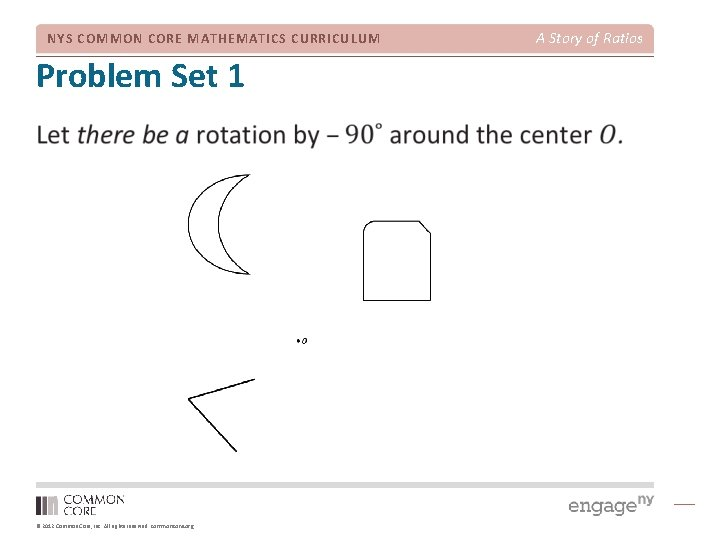NYS COMMON CORE MATHEMATICS CURRICULUM Problem Set 1 © 2012 Common Core, Inc. All rights reserved. commoncore. org A Story of Ratios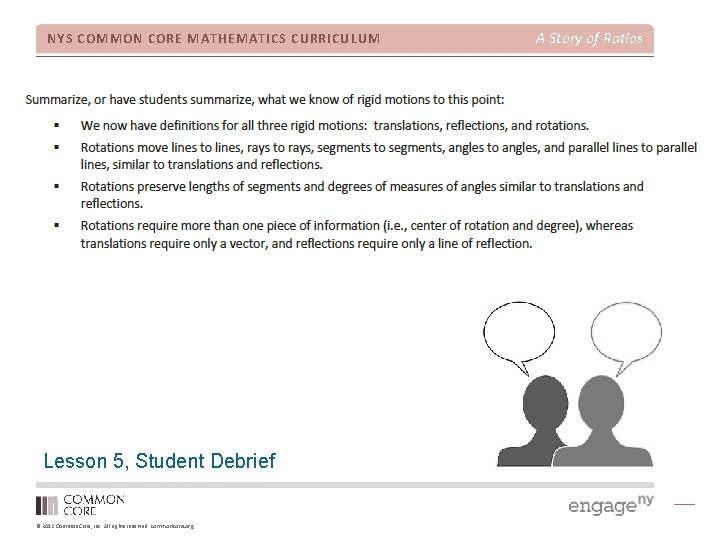NYS COMMON CORE MATHEMATICS CURRICULUM Lesson 5, Student Debrief © 2012 Common Core, Inc. All rights reserved. commoncore. org A Story of RatiosNYS COMMON CORE MATHEMATICS CURRICULUM A Story of Ratios L 6: Rotations of 180 Degrees • Students know that a point (a, b) on the coordinate plane, after a rotation of 180˚ will be located at (-a, -b). • The point, center, and image of point are collinear. Lesson 6, Concept Development © 2012 Common Core, Inc. All rights reserved. commoncore. org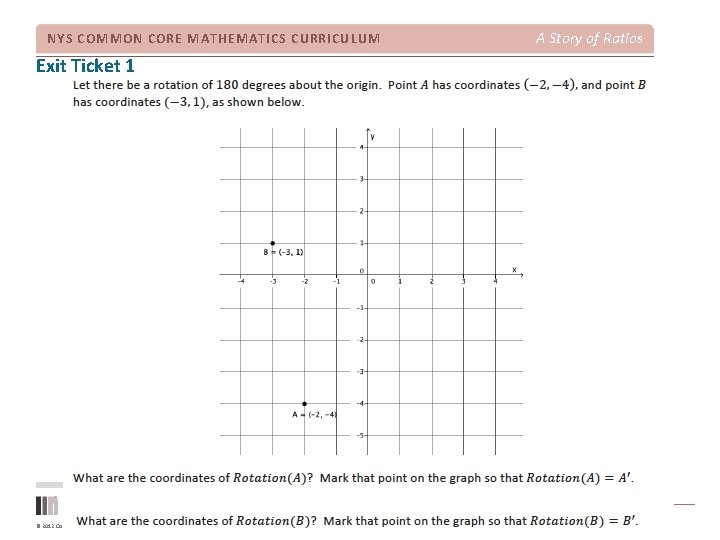NYS COMMON CORE MATHEMATICS CURRICULUM Exit Ticket 1 © 2012 Common Core, Inc. All rights reserved. commoncore. org A Story of Ratios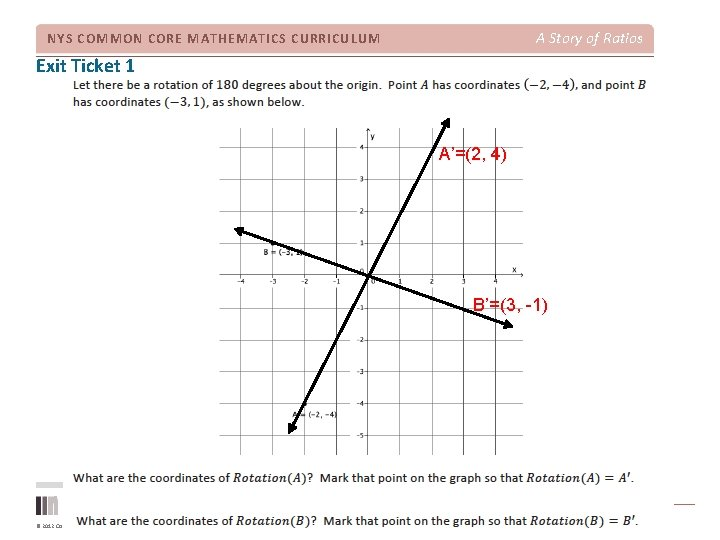A Story of Ratios NYS COMMON CORE MATHEMATICS CURRICULUM Exit Ticket 1 A’=(2, 4) B’=(3, -1) © 2012 Common Core, Inc. All rights reserved. commoncore. org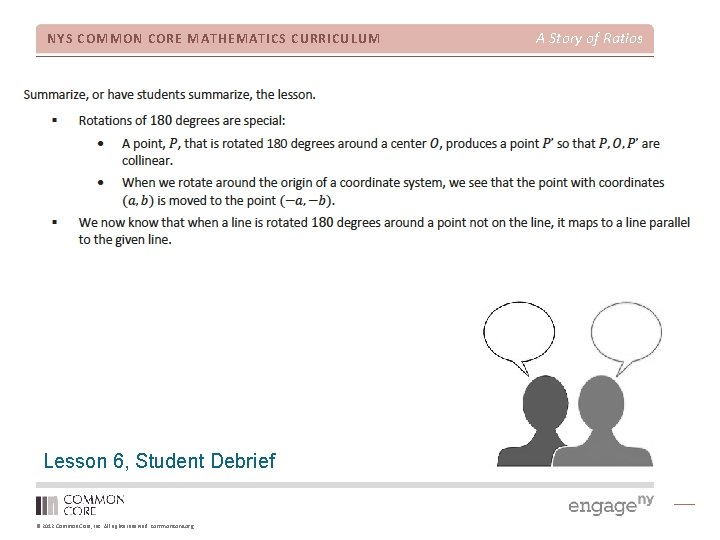NYS COMMON CORE MATHEMATICS CURRICULUM Lesson 6, Student Debrief © 2012 Common Core, Inc. All rights reserved. commoncore. org A Story of Ratios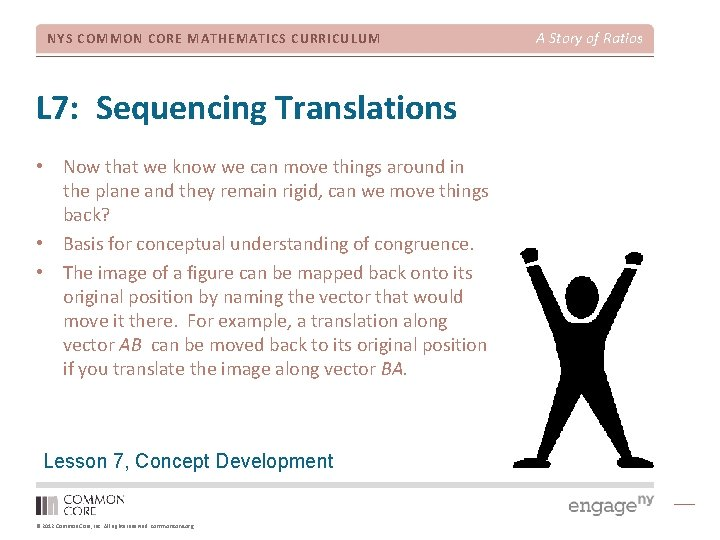NYS COMMON CORE MATHEMATICS CURRICULUM L 7: Sequencing Translations • Now that we know we can move things around in the plane and they remain rigid, can we move things back? • Basis for conceptual understanding of congruence. • The image of a figure can be mapped back onto its original position by naming the vector that would move it there. For example, a translation along vector AB can be moved back to its original position if you translate the image along vector BA. Lesson 7, Concept Development © 2012 Common Core, Inc. All rights reserved. commoncore. org A Story of Ratios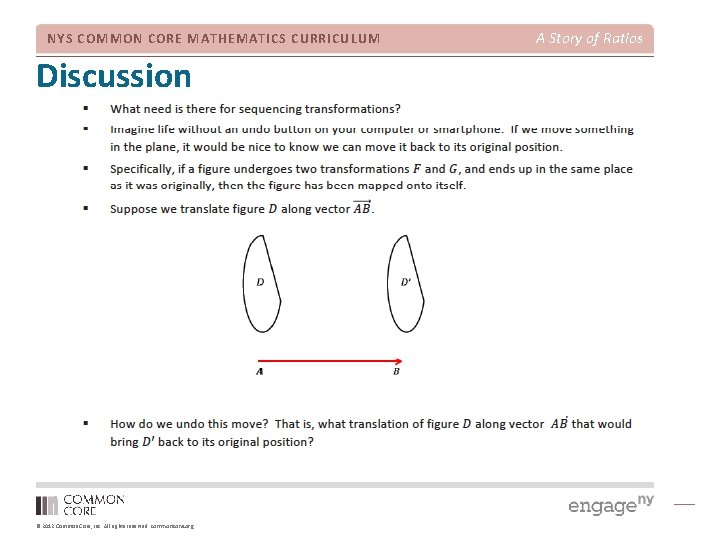NYS COMMON CORE MATHEMATICS CURRICULUM Discussion © 2012 Common Core, Inc. All rights reserved. commoncore. org A Story of Ratios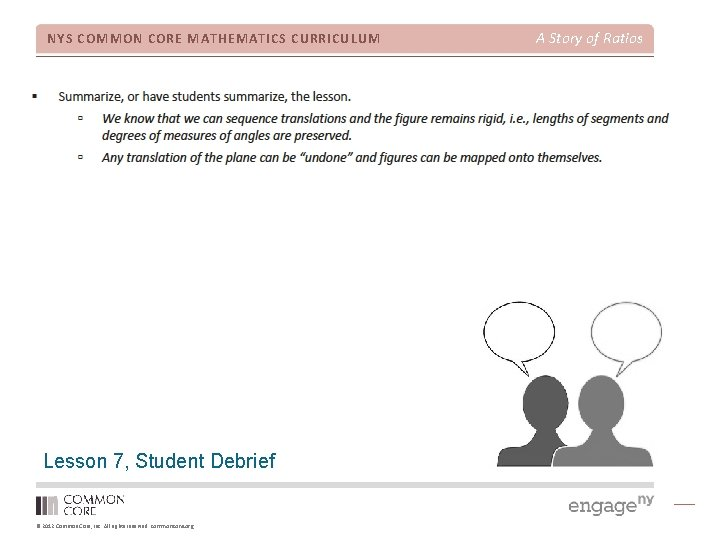NYS COMMON CORE MATHEMATICS CURRICULUM Lesson 7, Student Debrief © 2012 Common Core, Inc. All rights reserved. commoncore. org A Story of RatiosNYS COMMON CORE MATHEMATICS CURRICULUM L 8: Sequencing Reflections and Translations • A figure has been translated along a vector and reflected across a line. Will its image be in the same place if the figure had been reflected first and translated second? • The order in which we perform our basic rigid motions has an effect on the final location of an image. • Supports the development of conceptual understanding of congruence. Lesson 8, Concept Development © 2012 Common Core, Inc. All rights reserved. commoncore. org A Story of RatiosNYS COMMON CORE MATHEMATICS CURRICULUM Discussion © 2012 Common Core, Inc. All rights reserved. commoncore. org A Story of RatiosNYS COMMON CORE MATHEMATICS CURRICULUM Discussion © 2012 Common Core, Inc. All rights reserved. commoncore. org A Story of RatiosNYS COMMON CORE MATHEMATICS CURRICULUM Lesson 8, Student Debrief © 2012 Common Core, Inc. All rights reserved. commoncore. org A Story of Ratios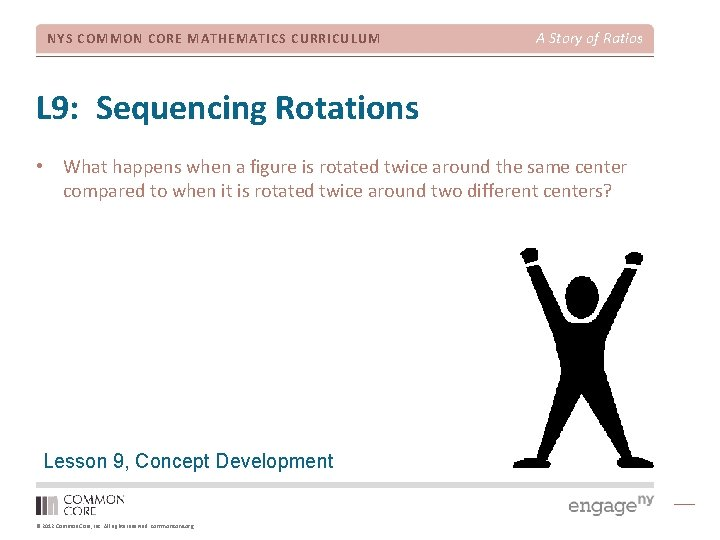NYS COMMON CORE MATHEMATICS CURRICULUM A Story of Ratios L 9: Sequencing Rotations • What happens when a figure is rotated twice around the same center compared to when it is rotated twice around two different centers? Lesson 9, Concept Development © 2012 Common Core, Inc. All rights reserved. commoncore. org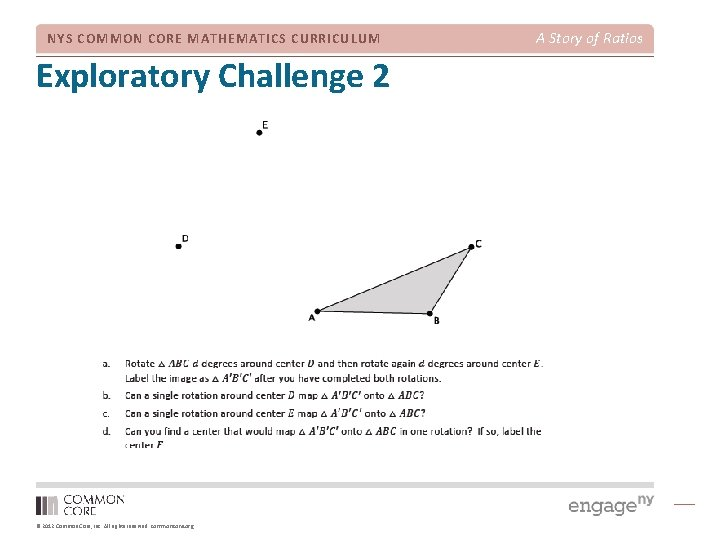NYS COMMON CORE MATHEMATICS CURRICULUM Exploratory Challenge 2 © 2012 Common Core, Inc. All rights reserved. commoncore. org A Story of Ratios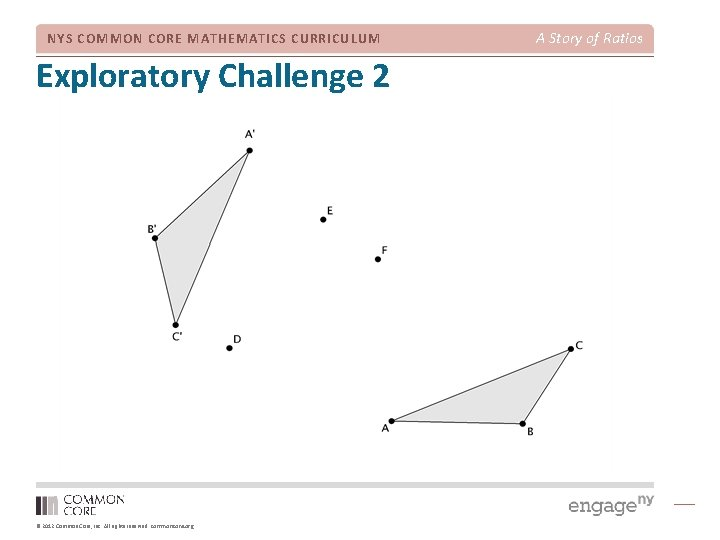NYS COMMON CORE MATHEMATICS CURRICULUM Exploratory Challenge 2 © 2012 Common Core, Inc. All rights reserved. commoncore. org A Story of RatiosNYS COMMON CORE MATHEMATICS CURRICULUM Lesson 9, Student Debrief © 2012 Common Core, Inc. All rights reserved. commoncore. org A Story of Ratios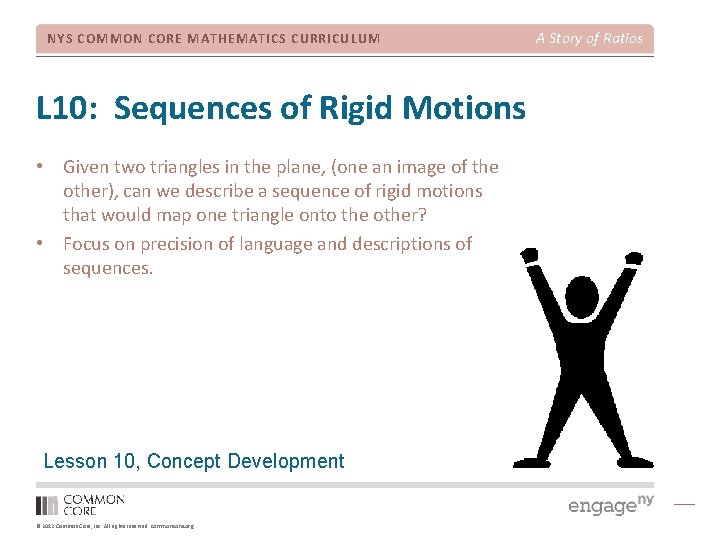NYS COMMON CORE MATHEMATICS CURRICULUM L 10: Sequences of Rigid Motions • Given two triangles in the plane, (one an image of the other), can we describe a sequence of rigid motions that would map one triangle onto the other? • Focus on precision of language and descriptions of sequences. Lesson 10, Concept Development © 2012 Common Core, Inc. All rights reserved. commoncore. org A Story of RatiosNYS COMMON CORE MATHEMATICS CURRICULUM Exercise 4 © 2012 Common Core, Inc. All rights reserved. commoncore. org A Story of RatiosNYS COMMON CORE MATHEMATICS CURRICULUM Lesson 10, Student Debrief © 2012 Common Core, Inc. All rights reserved. commoncore. org A Story of RatiosNYS COMMON CORE MATHEMATICS CURRICULUM A Story of Ratios L 11: Definition of Congruence and Some Basic Properties • Congruence is defined as a sequence of basic rigid motions that maps one figure onto another. • A congruence enjoys the same properties as the individual basic rigid motions. Lesson 11, Concept Development © 2012 Common Core, Inc. All rights reserved. commoncore. orgNYS COMMON CORE MATHEMATICS CURRICULUM Exercise 1 © 2012 Common Core, Inc. All rights reserved. commoncore. org A Story of Ratios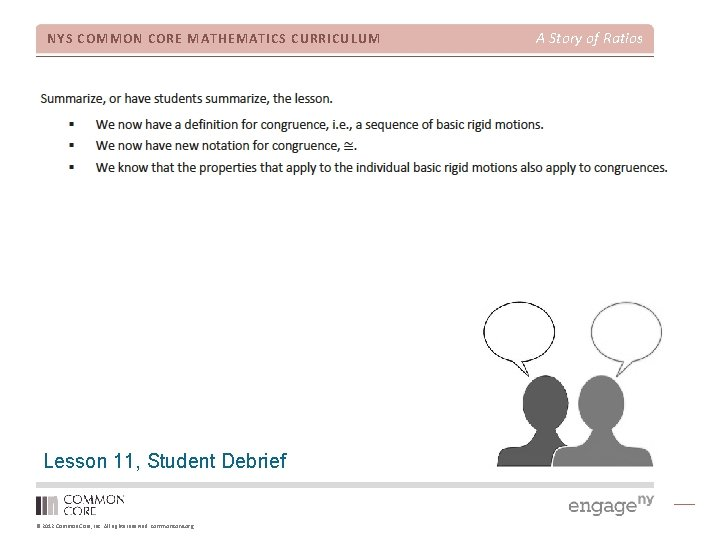NYS COMMON CORE MATHEMATICS CURRICULUM Lesson 11, Student Debrief © 2012 Common Core, Inc. All rights reserved. commoncore. org A Story of Ratios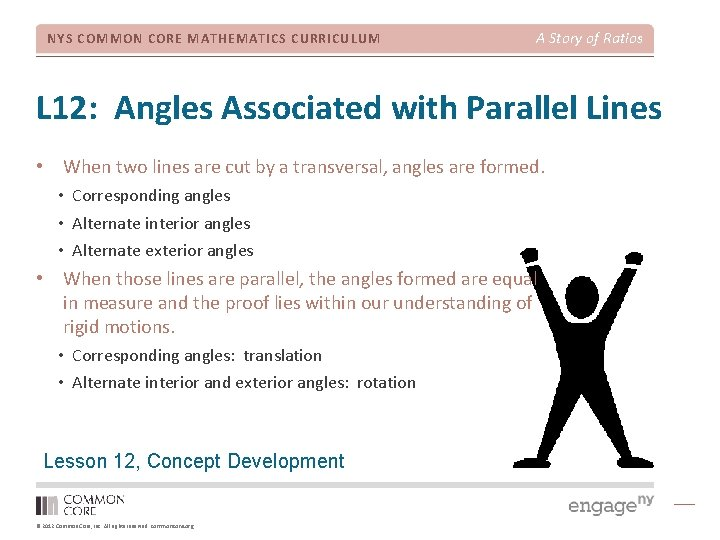NYS COMMON CORE MATHEMATICS CURRICULUM A Story of Ratios L 12: Angles Associated with Parallel Lines • When two lines are cut by a transversal, angles are formed. • Corresponding angles • Alternate interior angles • Alternate exterior angles • When those lines are parallel, the angles formed are equal in measure and the proof lies within our understanding of rigid motions. • Corresponding angles: translation • Alternate interior and exterior angles: rotation Lesson 12, Concept Development © 2012 Common Core, Inc. All rights reserved. commoncore. org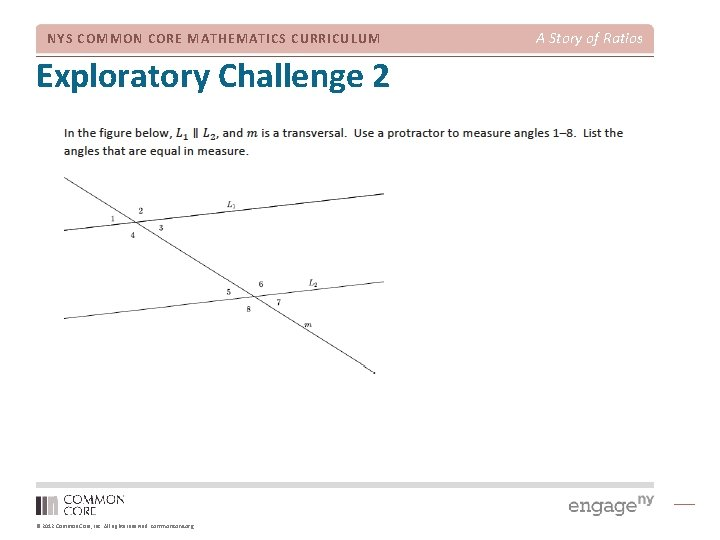NYS COMMON CORE MATHEMATICS CURRICULUM Exploratory Challenge 2 © 2012 Common Core, Inc. All rights reserved. commoncore. org A Story of RatiosNYS COMMON CORE MATHEMATICS CURRICULUM A Story of Ratios Exploratory Challenge 2 The angles are equal in measure. A translation along the transversal will map one angle onto the other. The angles are equal in measure. A rotation around the midpoint of the segment of the transversal between the parallel lines will map one angle onto the other. © 2012 Common Core, Inc. All rights reserved. commoncore. org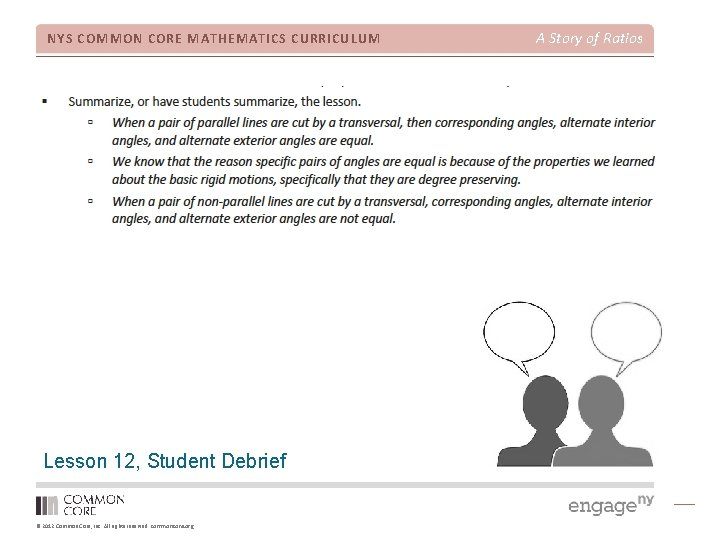NYS COMMON CORE MATHEMATICS CURRICULUM Lesson 12, Student Debrief © 2012 Common Core, Inc. All rights reserved. commoncore. org A Story of Ratios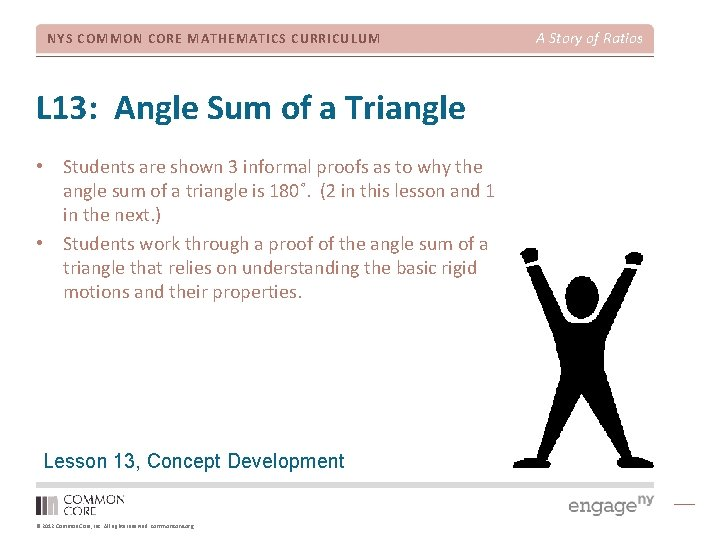NYS COMMON CORE MATHEMATICS CURRICULUM L 13: Angle Sum of a Triangle • Students are shown 3 informal proofs as to why the angle sum of a triangle is 180˚. (2 in this lesson and 1 in the next. ) • Students work through a proof of the angle sum of a triangle that relies on understanding the basic rigid motions and their properties. Lesson 13, Concept Development © 2012 Common Core, Inc. All rights reserved. commoncore. org A Story of Ratios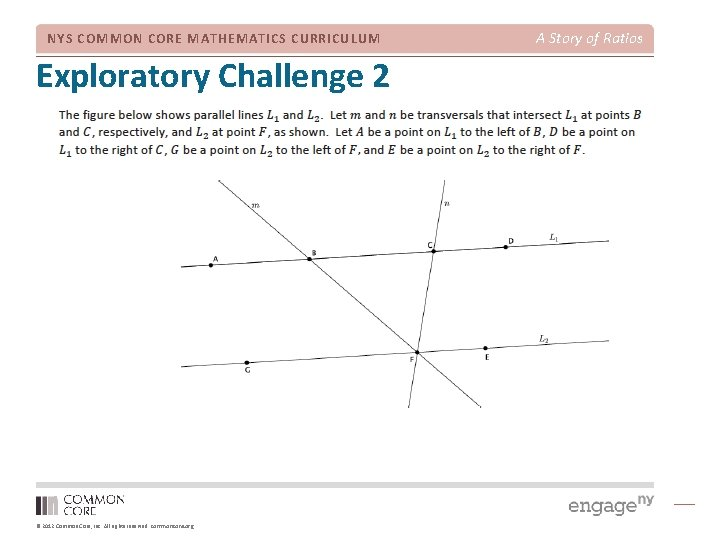NYS COMMON CORE MATHEMATICS CURRICULUM Exploratory Challenge 2 © 2012 Common Core, Inc. All rights reserved. commoncore. org A Story of RatiosNYS COMMON CORE MATHEMATICS CURRICULUM Lesson 13, Student Debrief © 2012 Common Core, Inc. All rights reserved. commoncore. org A Story of Ratios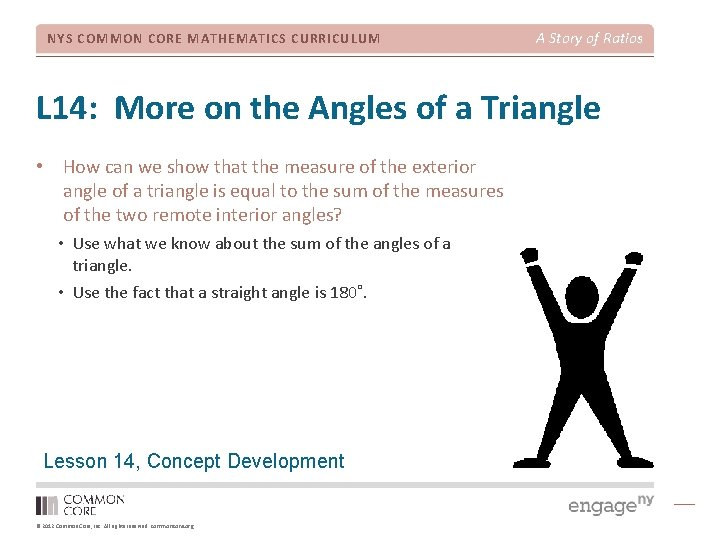NYS COMMON CORE MATHEMATICS CURRICULUM A Story of Ratios L 14: More on the Angles of a Triangle • How can we show that the measure of the exterior angle of a triangle is equal to the sum of the measures of the two remote interior angles? • Use what we know about the sum of the angles of a triangle. • Use the fact that a straight angle is 180˚. Lesson 14, Concept Development © 2012 Common Core, Inc. All rights reserved. commoncore. org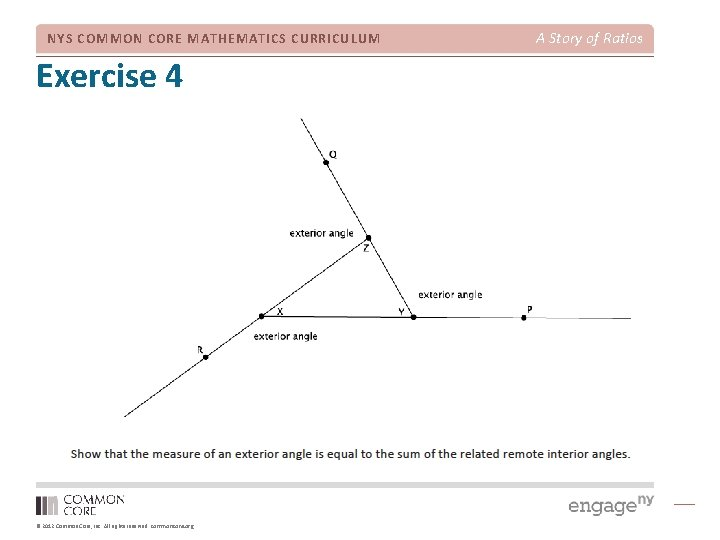NYS COMMON CORE MATHEMATICS CURRICULUM Exercise 4 © 2012 Common Core, Inc. All rights reserved. commoncore. org A Story of Ratios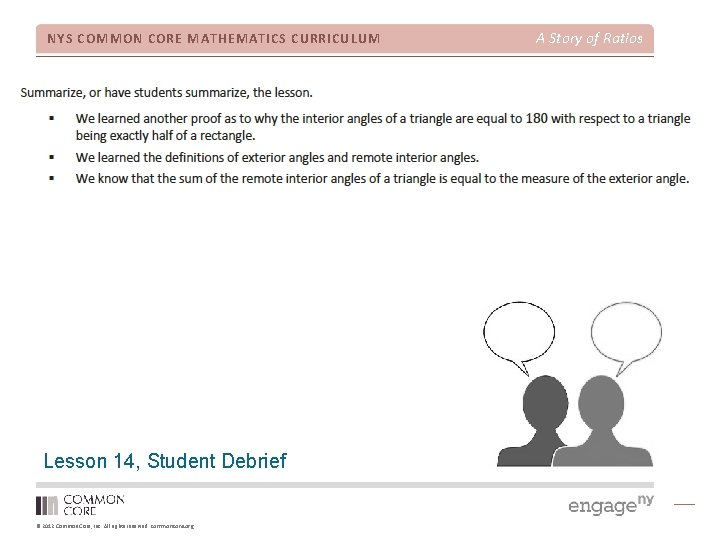NYS COMMON CORE MATHEMATICS CURRICULUM Lesson 14, Student Debrief © 2012 Common Core, Inc. All rights reserved. commoncore. org A Story of RatiosNYS COMMON CORE MATHEMATICS CURRICULUM A Story of Ratios L 15: Informal Proof of the Pythagorean Theorem • Square within a square proof. • Applies knowledge learned in Module 1 with respect to the first Law of Exponents. • Students use what they know about congruence to verify that four right triangles would have equal areas and apply properties of congruence to make claims about angle sums. • Students know how to determine the measure of an unknown angle of a straight angle. Lesson 15, Concept Development © 2012 Common Core, Inc. All rights reserved. commoncore. org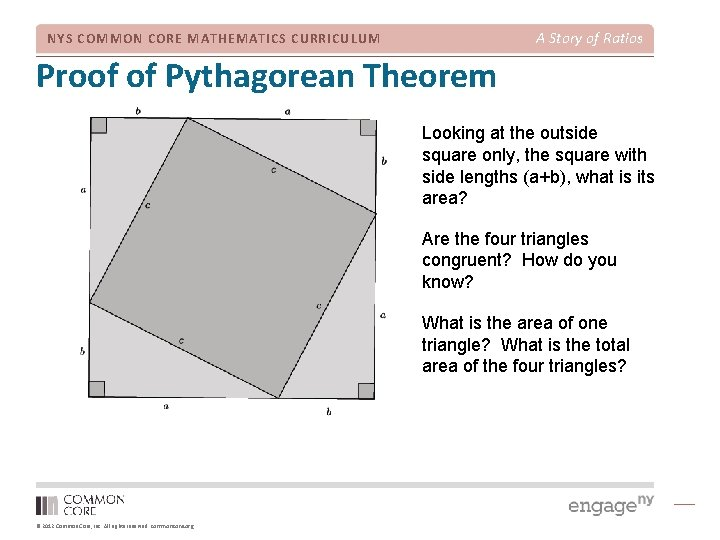A Story of Ratios NYS COMMON CORE MATHEMATICS CURRICULUM Proof of Pythagorean Theorem Looking at the outside square only, the square with side lengths (a+b), what is its area? Are the four triangles congruent? How do you know? What is the area of one triangle? What is the total area of the four triangles? © 2012 Common Core, Inc. All rights reserved. commoncore. org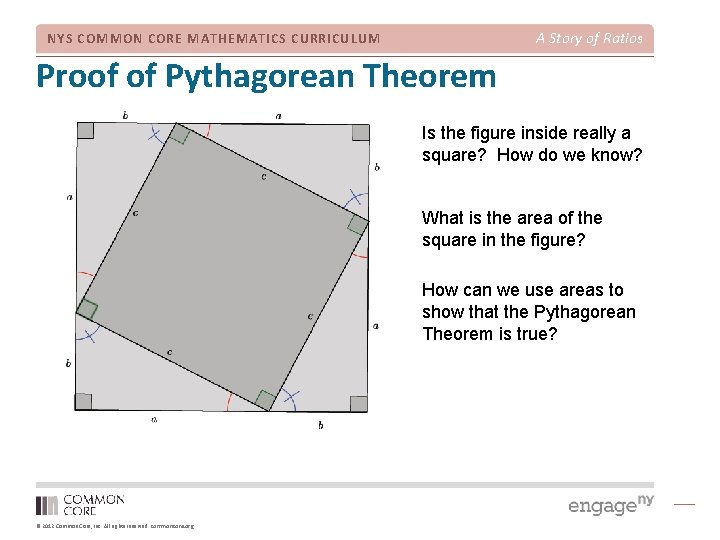A Story of Ratios NYS COMMON CORE MATHEMATICS CURRICULUM Proof of Pythagorean Theorem Is the figure inside really a square? How do we know? What is the area of the square in the figure? How can we use areas to show that the Pythagorean Theorem is true? © 2012 Common Core, Inc. All rights reserved. commoncore. org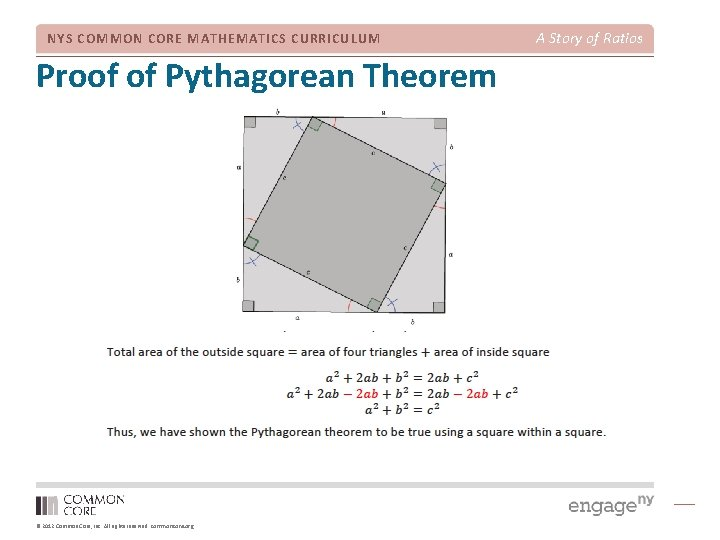NYS COMMON CORE MATHEMATICS CURRICULUM Proof of Pythagorean Theorem © 2012 Common Core, Inc. All rights reserved. commoncore. org A Story of Ratios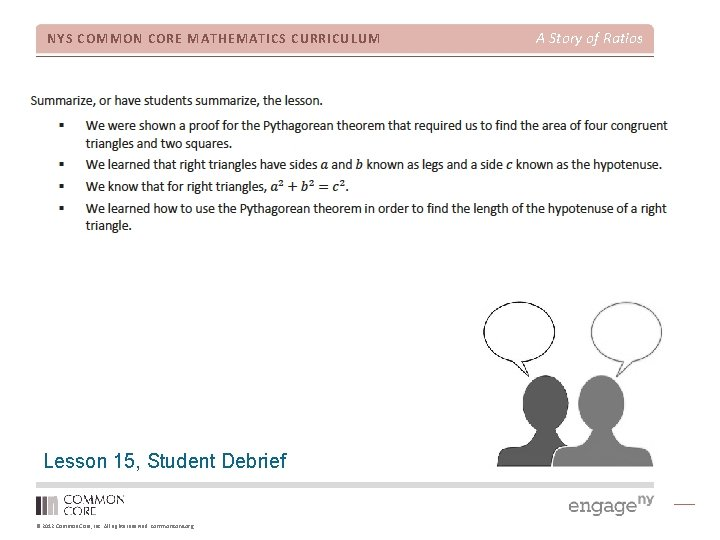NYS COMMON CORE MATHEMATICS CURRICULUM Lesson 15, Student Debrief © 2012 Common Core, Inc. All rights reserved. commoncore. org A Story of Ratios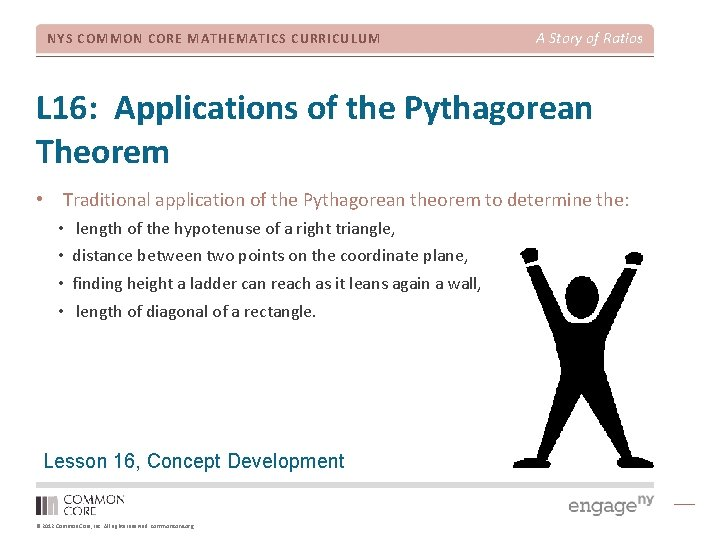NYS COMMON CORE MATHEMATICS CURRICULUM A Story of Ratios L 16: Applications of the Pythagorean Theorem • Traditional application of the Pythagorean theorem to determine the: • • length of the hypotenuse of a right triangle, distance between two points on the coordinate plane, finding height a ladder can reach as it leans again a wall, length of diagonal of a rectangle. Lesson 16, Concept Development © 2012 Common Core, Inc. All rights reserved. commoncore. orgNYS COMMON CORE MATHEMATICS CURRICULUM Exercise 3 © 2012 Common Core, Inc. All rights reserved. commoncore. org A Story of RatiosNYS COMMON CORE MATHEMATICS CURRICULUM Lesson 16, Student Debrief © 2012 Common Core, Inc. All rights reserved. commoncore. org A Story of RatiosNYS COMMON CORE MATHEMATICS CURRICULUM Agenda Introduction to the Module Concept Development Module Review © 2012 Common Core, Inc. All rights reserved. commoncore. org A Story of RatiosNYS COMMON CORE MATHEMATICS CURRICULUM Biggest Takeaway Turn and Talk: • What questions were answered for you? • What new questions have surfaced? © 2012 Common Core, Inc. All rights reserved. commoncore. org A Story of RatiosNYS COMMON CORE MATHEMATICS CURRICULUM A Story of Ratios Key Points • Experimental verifications of the basic rigid motions. • Precision of language in descriptions of sequences. • Immediate application of concept of congruence for understanding angle relationships. • Application of congruence and angle relationships to explain a proof of the Pythagorean theorem. © 2012 Common Core, Inc. All rights reserved. commoncore. org